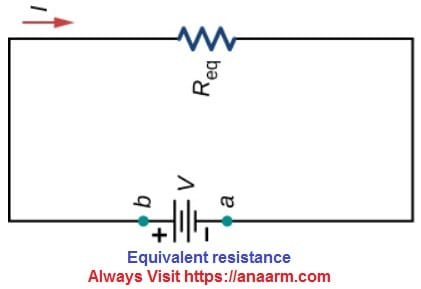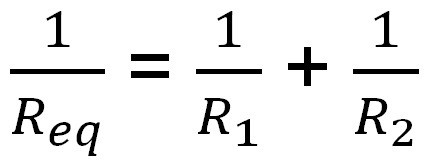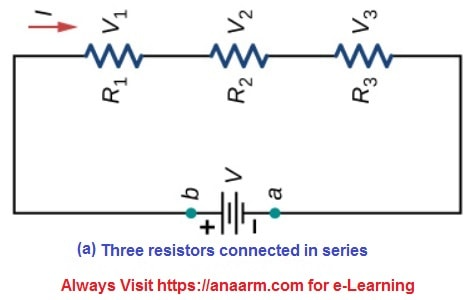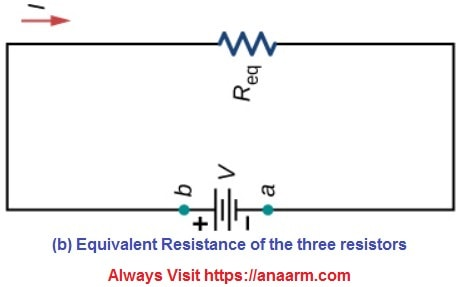# SS One Physics Third Term

## Announcement for Students in: SS One, SS Two & SS Three, Third Term

Study Online & Get
Better SSCE (WAEC & NECO) Results
Than in Tutorial Classes
Tutorial or Home lesson may not be so effective in your quest for academic excellence, especially when you have the opportunity to access our content-rich, well-formatted e-Notes, Video Lessons, Voice Notes, etc

#### Week 1 Introduction to Current Electricity Take Online Quiz Here

What is an electric circuit? It is a complete conducting loop that provides a path for the flow of electric charges (or electrons). As shown in Figure 1 below, [sociallocker id=”8247″] the battery, switch, resistor and the wire form a closed loop, thus creating a path for current (or electron) to flow. Figure 2 shows the actual connection of the components that make up a simple electric circuit.Figure 1Figure 2

[/sociallocker] What follows after a good understanding of electric circuit is Ohm’s law. Ohm’s law is stated as follows:

V = IR

where V = voltage, I = current, and R = resistance.

Ohm’s law allows you to determine the characteristics of a circuit, such as how much current is flowing through it, if you know the voltage of the battery in the circuit and how much resistance is in the circuit.

Define Ohm’s Law: Ohm’s Law states that the current flowing in a conductor is directly proportional to the applied potential difference provided the temperature of the conductor and other physical properties such as area and length remain constant. Watch the video on Ohm’s LawEvaluation
1. Explain how to connect an ammeter in a circuit.
2. Explain how to connect a voltmeter in circuit.
3. What component provides energy in a DC circuit? Mention the type of energy.

#### Week 2 Ohm’s law, resistors in circuits, electrical energy and power Check Textbooks & References Here

Sub topics:
Resistors and cells in series
Resistors and cells in parallel

#### Resistors in ParallelWhen resistors are connected in parallel, they have the same potential difference across them. In order to calculate the equivalent resistance of two resistors connected in parallel, the equation below is used.Questions Derive an expression for the resistance of two conductors connected in: (i) series (ii) parallel. (Farinde pg 101 Q9)

#### Resistors in ParallelWhen resistors are connected in parallel, they have the same potential difference across them. In order to calculate the equivalent resistance of two resistors connected in parallel, the equation below is used.Questions Derive an expression for the resistance of two conductors connected in: (i) series (ii) parallel. (Farinde pg 101 Q9)

#### Week 3 Resistors and Cells in Series and Parallel Go to Other Classes and Terms Here

Sub topics
Resistors in series
Resistors in parallel
Cells in series
Celle in parallel

The following video explains how resistors are connected in series and parallel.

#### Resistors in Series

The total resistance (or equivalent resistance) in a circuit in which resistors are connected in series is equal to the sum of the individual resistances.

i.e.       Req = R1 + R2 + R3 —- for 3 resistors

Req = R1 + R2 + R3 + … + Rn —- for n resistors

Also, if a current, I, is applied at one end of a group of resistors connected in series, it would flow through each resistor sequentially to the opposite end.#### Resistors in ParallelWhen resistors are connected in parallel, they have the same potential difference across them. In order to calculate the equivalent resistance of two resistors connected in parallel, the equation below is used.Questions Derive an expression for the resistance of two conductors connected in: (i) series (ii) parallel. (Farinde pg 101 Q9)

Work in Progress

Work in Progress

Work in Progress

#### Week 7 Mid-Term Revision and Test Watch Videos on Any Lesson Here

Take a Quiz on SS1 Physics 3rd Term
Coming Soon

Jump to Any Week Here Week 1 Week 2 Week 3 Week 4 Week 5 Week 6 Week 7 Week 8 Week 9 Week 10 School Resumption Update

## Senior School e-Classes

View Subjects/Courses
Browse SSS Scheme of Work
 Browse by Term & Class 1st SS 1 SS 2 SS 3 2nd SS 1 SS 2 SS 3 3rd SS 1 SS 2 SS 3

SS One Subjects 1st Term

SS Two Subjects 1st Term

SS Three Subjects 1st Term

NTI PgDE Past Q & A

SS One Subjects 2nd Term

SS Two Subjects 2nd Term

SS Three Subjects 2nd Term

Textbooks and References

SS One Subjects 3rd Term

SS Two Subjects 3rd Term

SSCE Past Questions

TRCN Past Q & A